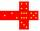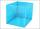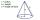# Cube edge

Determine the edges of the cube when the surface is equal to 37.5 cm square.

Result

a =  2.5 cm

#### Solution:Leave us a comment of example and its solution (i.e. if it is still somewhat unclear...):Be the first to comment!## Next similar examples:

1. CubeHow many times increases the surface area of a cube with edge 23.4 cm if the length of the edge doubled?
2. Cube 2How many times will increase if the surface area of the cube if we triple length of its edge?
3. The cubeThe surface of the cube is 150 square centimeters. Calculate: a- the content of its walls b - the length of its edges
4. Cube 5The surface of the cube is 15.36 dm2. How will change the surface area of this cube if the length of the edges is reduced by 2 cm?
5. Cube edgesThe sum of the lengths of the cube edges is 42 cm. Calculate the surface of the cube.
6. CubeThe sum of all cube edges is 30cm. Find the surface area of the cube.
7. Cube surface areaWall of cube has content area 99 cm square. What is the surface of the cube?
8. The cube - simpleCalculate the surface of the cube measuring 15 centimeters.
9. Unknown number 11That number increased by three equals three times itself?
10. AlleyAlley measured a meters. At the beginning and end are planted poplar. How many we must plant poplars to get the distance between the poplars 15 meters?
11. ExpressionSolve for a specified variable: P=a+4b+3c, for a
12. SimplifySimplify the following problem and express as a decimal: 5.68-[5-(2.69+5.65-3.89) /0.5]
13. HotelThe hotel has a p floors each floor has i rooms from which the third are single and the others are double. Represents the number of beds in hotel.
14. Two machinesPerformances of two machines are in a ratio of 7:12. A machine with less power produced 406 pieces of products per shift. a) How many pieces produced per shift second machine? b) How many pieces produced two machines together for five shifts?
15. Negative in equation2x + 3 + 7x = – 24, what is the value of x?
16. Dropped sheetsThree consecutive sheets dropped from the book. The sum of the numbers on the pages of the dropped sheets is 273. What number has the last page of the dropped sheets?
17. The diagramThe diagram is a cone of radius 8cm and height 10cm. The diameter of the base is. ..